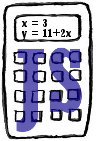## Posts

Showing posts from December, 2012

### CalcJS: Evaluating complex math expressions in JavaScript in 120 linesSometimes it’s useful to express our math thought more explicitly, so instead of saying: x = 10 * 1.1 * 1.3 we’d prefer to say: cost = 10, price = cost * 1.1, RRP = price * 1.3 . CalcJS helps you to evaluate complex math expressions described in human readable format. Couple of days ago I’ve seen an interesting code snippet of a JavaScript evaluator. I thought the idea is great so I started to experiment: how hard would it be to create a framework that can evaluate complex math expressions that can use variables? Humans usually express the math expressions in infix format, so I needed to create a simple stack based evaluator that is able to parse infix formulas. The algorithm I used was the Shunting-yard algorithm that was invented by Dijsktra (like so many other great algorithms). The basic idea is to use two stacks, one for values and one for operators. When we see an operator that’s precedence is smaller than the previous (like + following a *), we evaluate what we'